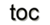Next: 5.3 Data Structure Storage Up: 5. Application Concepts Previous: 5.1 Generic Data Structures

# 5.2 Boundary Operation

The concept of chains transforms the properties of a cell complex directly into a computationally manageable algebraic structure. The practical concepts in the subsequent sections use these mechanisms to derive different relations between the cells, such as incidence, adjacence, and boundary operations. The essential requirement for a generic data structure implementation of a cell complex is to satisfy the homological concepts of a chain in a computational environment and thereby the concept of the boundary operator thus obtaining, for a complex of dimension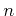, the sequence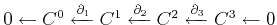. The complete chain complex of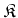is defined by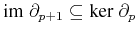, or less abstractly,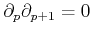. This is of particular interest because, while, in general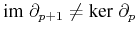and the part of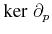not in the inclusion contains useful information .

The given boundary operator, introduced in Section 1.4.1, lacks generality, because the cell topology (see Section 1.3.2 for further details) can be arbitrarily complex, e.g., the given boundary homomorphism already has to be extended if a cube cell is used instead of a simplex cell. Therefore, the given poset notation of the cell topology is used to introduce a more general boundary mechanism which can be easily converted into a computationally efficient operation. The boundary operator can then be used to traverse the levels of the poset, e.g., a three-dimensional simplex, illustrated in Figure 5.8.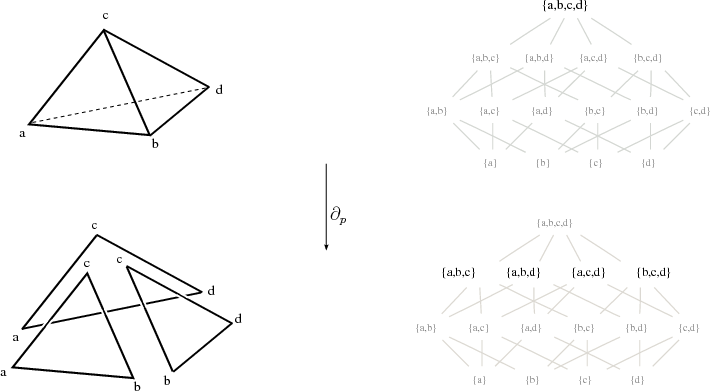As can be seen, the boundary operator simply decreases the level of evaluation of the cell topology poset. The boundary operator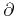transforms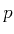-chains into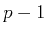-chains, which is compatible with the addition and external multiplication of chains. By the linearity property of the boundary operator, a unique and simple way of consistently deriving the boundary of a chain is given by: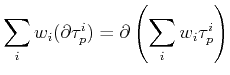(5.1)Next: 5.3 Data Structure Storage Up: 5. Application Concepts Previous: 5.1 Generic Data Structures

R. Heinzl: Concepts for Scientific Computing